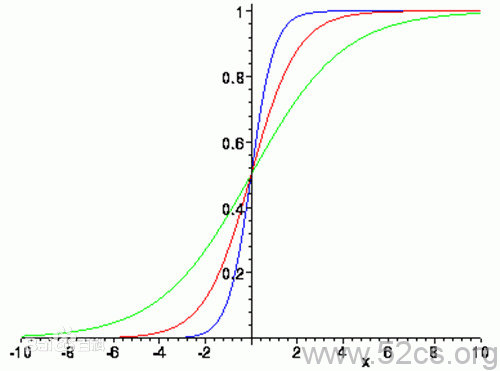# Logit models, or Logistic regression1. Logistic function

Logistic曲线  (先把为什么叫Logistic这个名字抛在一边) 从函数角度讲，就是从实数定义域  到值域  的单调映射。





 就是logistic分布函数:





2. Logistic distribution function

Logistic曲线  的性质：

• 从实数映射到[0,1]区间，
•  单调（上升），



• 神经网络中，它表示激活函数时，记号常用  ,
• 类比  代表标准正态分布函数一样，实际上 Probit 模型与 Logit 模型不同之处就在于此。

3. Logit model, or Logistic regression

logit model 就是 Logistic regression
logit  表示的意思是对数几率：







Logit models是说（  是数据矩阵，  是参数向量）：



（即概率的对数几率是线性模型）



（即概率本身是Logistic分布函数）

• 所以logistic 就是logit 的形容词，它从（统计）命名上来看，与逻辑logic没有任何关系；尽管它最初用于二元0-1分类，会让人产生将Logistic和logic联系起来的直觉反应，尤其是学计算机系的。
• 我的看法：在知道这个统计命名事实的基础上，可以将logic和Logistic联系起来，就像PageRank后来被人解释为Larry Page一样。并且这样的联系还很容易记住这个事实：Logistic regression是分类器，虽然它有回归二字。
• 为什么叫regression : 线性回归的两个扩展。（详见参考文献2第1章）
• 我的看法 : 1）它是通过计算概率用于分类的，所以叫做回归也可以。2）线性回归  ，而logit model对概率取对数几率后  ，通过引入隐变量  ，可将线性regression的误差项和logit模型的隐变量项对应起来。（详见参考文献1第7章）

1. David A. Freedman (2009). Statistical Models: Theory and Practice.
• “Logistic regression is a synonym for logit models.”见P128
• 隐变量  ，见第七章。

2.  Kevin P. Murphy (2012). Machine learning: A Probabilistic Perspective.

• "This is called logistic regression due to its similarity to linear regression (although it is a form of classification, not regression!)."   见P21.